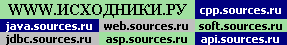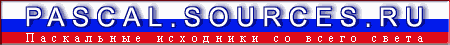Powered byНа Главную Pascal Форум Информер Страны мира
Графика    >>    plotterPlotter - Plot F(x,y) Function Graphic for BP7 Al Goldberg 17.05.1991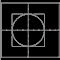Объяснение принципов преобразования реальных координат в пиксельные координаты на экране. Приведена программа, рисующая график. Для запуска требуется EGAVGA.BGI v2.0. PLOTTER contains a methodical scheme to set up an analytic coordinate system inside a TP graphics window and also has the functions u(x), v(y) that transform local analytic x,y coordinates to pixels, thus allowing the use of analytic algebra entirely in local x,y coordinates. EGAVGA.BGI required.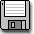6k```PLOTTER.PAS 05/16/91 Al Goldberg, 47 Nancy Rd., Newton, Ma 02167 ---------------------------------------------------------------- It is awkward to make analytic plots in a Turbo Pascal graphics window because analytic functions (reals) generally have an origin near the bottom left and are positive towards the top right while TP's graphic system expects XY coordinates to be given as pixels (integers) with origin at top left and grow positively towards the bottom right. There is also a problem with scale lengths since one would want to assign unit values in a window that might differ from the preset pixel-numbers. Finally there can be a problem with linearity caused by rectangular screen shapes. In order to handle these difficulties PLOTTER contains a methodical scheme to set up an analytic coordinate system inside a TP graphics window and also has the functions u(x), v(y) that transform local analytic x,y coordinates to pixels, thus allowing the use of analytic algebra entirely in local x,y coordinates. Similarly, lines, labels, circles, and so on can be located in the x,y system but drawn with standard TP graphics methods. Call pixel coordinates u,v with an origin at top left that increase to uu,vv at bottom right. u,v's are integer-type. Define an analytic coordinate system of reals with origin at the bottom left corner that increase to top right; call these absolute analytic coordinates, x',y'. Also define real-type local analytic coordinates x,y whose origin is arbitrary - it may be anywhere in the window or may be outside it. In local coordinates the abs origin is point x0,y0, the point at top right is x1,y1, and any other point is x,y from the local origin. For simplicity take the local origin to be outside the window, below and to the left of the abs origin. In abs coordinates, then, a local point x,y is x' = x - x0, y' = y - y0, and the screen is xx = x1 - x0 wide by yy = y1 - y0 high in either of the analytic coordinates. To define the system, specify values for points x0,y0 and x1,y1; if the local origin is inside the window then x0,y0 will have negative values. Conversion from abs to pixel coordinates is u = uu*x'/xx and v = vv - vv*y'/yy. With conversion from real to integer the functions can be defined: u(x) = Trunc(uu * (x - x0)/xx) v(y) = vv - Trunc(vv * (y - y0)/yy) where u(x) & v(y) return integer-types and x's & y's are real-types. Linearity can be a problem when graphic figures are generated from analytic expressions rather than TP's built-in functions for circles, arcs, or the like. True linearity occurs only when the coordinate system gives equal physical lengths for equal unit distances in both x and y directions. Thus a square of, say, 5 units on a side ought to have identical sides when measured by a ruler on the screen. Not only should the coordinate system be so defined, but there are two other elements that need to be considered: the logical shape of the screen as given by pixel dimensions of the graphics card in use - 720x348 Hercules or 640x480 VGA, for example - and the physical dimensions of the video screen. The latter can vary substantially depending on the type of screen in use, as with a CRT monitor or elongated laptop LCD. It's also worth mentioning that although the reasoning here is to produce a linear screen, linearity isn't always needed nor desireable as it reduces the range of a plot. A sine function, for example, is recognizable when x & y scale factors differ while a circle looks very odd unless the two scales are identical. TP uses the term aspect ratio but doesn't really define it, although GetAspectRatio returns parameters by which an aspect ratio may be calculated and SetAspectRatio allows it to be changed. The following is taken from a manual on the C language which seems to use the term analogously: aspect ratio = (width/height) * (vv/uu), where the first term is obtained by physical measurement of the active portion of the screen, the second defined by pixel counts from GetMaxX & GetMaxY or, as mentioned below, by dimensions of a defined viewport. However, since TP only has information about the pixel values there could be defaults for the width/height term which may not always be correct. In any event TP does make internal compensation for screen shape in its graphics functions, and in the case of both my desktop CRT monitor (width/height = 1.550, 720x348 screen) and laptop LCD (1.316, 640x200) manages to produce fairly good circles on each, although probably there are cases where that isn't true. In the end it seems simpler to use information about screen shape in other ways. Thus, the functions defined as coordinate transforms, above, use uu,xx & vv,yy separately. Call phi = (width/height), a parameter that describes the shape of the active region of the screen and let us presume it can be determined by a ruler from physical dimensions of a rectangle drawn about the screen. We can compare that value to one computed from GetAspectRatio and (vv/uu), choosing in case of a discrepancy the one that makes best linearity. For reference, GetAspectRatio returns the parameters Xasp, Yasp, from which (Xasp/Yasp) gives TP's aspect ratio according to my C manual, and, by earlier reasoning must also include defaults to account for the shape of the physical screen. If those defaults match the real, measurable, phi then the relation (Xasp/Yasp) = phi * (vv/uu) should be true. When defining the system choose numbers for the points x0,y0, & x1,y1 that define a square region; this assures that units along both axes will be uniform. Noting, however, that due to the generally rectangular shape of most screens - phi larger than 1 - we should increase the length of the x-axis to account for the extra space. Take, then, (x1 - x0) = (y1 - y0)*phi, but, since eventually the terms for width and height, xx & yy, will be needed we can choose to specify the screen either by numeric values for both corners or for one corner and width, height. Analytic relations are needed for the missing parameters, either a corner not specified or width, height. In either event the condition that xx = yy*phi should be included in the specification. While the above discussion was based on a window the size of a full screen, there is nothing to prevent one from applying the same logic to a smaller area defined in SetViewPort. In that event the pixel system inside the port starts at 0,0 at top left and increases to uu,vv at the bottom right corner. All analytic coordinate relations apply to the newly defined space except that GetmaxX, GetmaxY still refer to the full screen, not to the newly defined port. It seems simplest to specify the port with pixel values referenced to the full screen; computed values for uu,vv of the port then are directly useable in an analytic system inside the port. PLOTTER.PAS does just this, and in a way that makes it easy to change the shape of the port so to observe what happens to TP's Circle and to a comparable one generated analytically.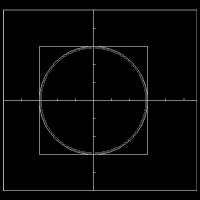PLOTTER.PAS is a program that demonstrates the ideas described here. It is intended as a prototype where coordinate parameters can be easily changed and from which various relevant parts may be copied to a 'working' program. Note the liberal use of a ruler in order to make sure that linearity is achieved, noting also that the writer has no scruples about fudging the value of phi until it does although he found TP's default parameters came fairly close to phi-measured on his screens. Note also that the standard documentation doesn't have much on how TP handles the aspect ratio. The writer would appreciate information in this area from anyone who cares to contribute.```